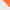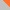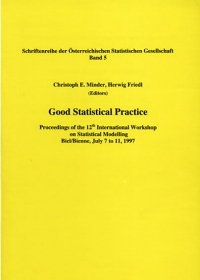Contact# Statistical Modelling Society

www.statmod.orgHomeThe SocietyThe Workshop The IWSM History of the IWSM ArchiveThe JournalNewsletterJoin the SocietyMembers OnlyContact Us

## Twelfth International Workshop on Statistical Modelling, Biel/Bienne, 1997, ProceedingsChristoph E. Minder, Herwig Friedl (Eds.)
Good Statistical Practice. Proceedings of the 12th International Workshop on Statistical Modelling. Biel/Bienne, July 7 to 11, 1997
(Schriftenreihe der Österreichischen Statistischen Gesellschaft, Band 5)
Wien: Österreichische Statistische Gesellschaft 1997
ISBN 3-7007-0046-6

## Contents

### Invited papers

Becher, H., K.Steindorf
Methods to analyse continuous covariables in epidemiological studies
Bednarski, T.
Frechet differentiability and robust statistical inference
Brant, L.J., G.N.Verbeke
Modelling longitudinal studies of aging
Davies, R.
Good statistical practice in data analysis for social sciences
Dodge, Y., J.Jureckova
Adaptive combination of tests
Draper, D.
Model uncertainty in "stochastic" and deterministic systems
Fahrmeir, L., Ch.Gieger
Is the rent for my flat too expensive?
Gelman, A., F.Y.Bois
How can statistical theory help with statistical practice? Example of a Bayesian analysis in toxicokinetics
Greenacre, M.
Data coding and visualization of social science data
Hampel, F.
Robust statistics and data analysis
Morgenthaler, St.
Robust conditional inference
Racine-Poon, A.
Good statistical practice in clinical development
Rendtel, U.
The analysis of the wage curve with panel data

### Contributed papers and Posters

Ambler, G., P.Royston
Models and model selection strategies with regression splines
Azizi, F., R.B.Davies
Mixed logistic regression models for longitudinal binary data: Representing frailty effects by variation in a base-line probability/dd>
Berghold, A., K.-D.Wernecke
Capture-recapture methods in the registration of congenital anomalies
Borhani Haghighi, A., R.B.Davies
The use of smoothing splines in random effect modelling of longitudinal data
Brewer, M.
Avoiding prior probabilities of guilt in forensic applications of graphical models
Caputo, A., A.Heinicke
What are the determinants for sociologists being satisfied with their job? - New insights by the application of graphical chain models
Chung, K.H., S.M.S.Lee
m/n bootstrap confidence regions
Colombi, R.
The marginal canonical association-multivariate logit model
Declerck, L., G.Molenberghs, M.Aerts
The influence of simplifying the Bahadur model on the likelihood ratio statistic
Dietz, E., D.Böhning
Interval estimating of mixture model parameters
Edlich, S.
A local estimating approach in multi-categorical varying-coefficient models
Eilers, P.H.C., B.D.Marx
Modelling with generalized additive structures and penalties
Ferreira, E., Núñez-Antón, V., Rodriguez-Póo, J.
Semiparametric approaches to signal extraction problems in economic time series: The case of the Spanish industrial production index
Fielding, A., N.Spencer
Modelling cost-effectiveness in general certificate of education A level: Some practical methodological innovations
Funck-Hüsges, C.
Generalized linear models with random effects and time varying coefficients
Gampe, J.
Spatial data analysis via smoothing spline models with correlated errors
Geys, H., G.Molenberghs, P.Williams
Analysis of clustered binary data with covariates specific to each observation
Graves, T.L., A.Karr, A.Mockus
Modeling software changes
Heuer, C.
Analysis of the Lexis diagram using multivariate adaptive regression splines (MARS)
Hutton, J.L.
Bias in meta-analysis with variable selection within studies
Kauermann, G., G.Tutz
Testing generalized linear models against smoothed alternatives
Krug, B.
Robust goodness-of-fit test in the Cox regression model
Lam, K.C., K.Lam
An alternative choice of dependent variable in neural network modeling for the generation of trading signals in a financial market
Lavergne, C., C.Trottier
From a conditional to a marginal point of view in GLM
Lee, Y.N., W.K.Li
On a double smooth transition time series model
Lischer, P.
The use of a Horowitz-like function for the analysis of chemical laboratory data
Manchester, L., K.Haller
Predicting cod stocks from air pressure fields: A problem in under determined regression
McDonald, J.W., P.W.F.Smith, J.J.Forster
Exact tests for log-linear models for mortality rates and standardized mortality ratios
Mezzetti, M., C.Robertson
A hierarchical Bayesian approach to age-specific backcalculation of cancer incidence rates
Mönkeberg, S., W.Stahel, R.Kegel, J.Staehelin
Factor analytic models for identifying sources of air pollution. Comparing graphical analyses and positive matrix factorization
Núñez-Antón, V., J.M. Rodriguez-Póo, P.Vieu
Longitudinal data with nonstationary errors: A nonparametric three-stage approach
Oldham, M.A., A.M.Sykes, A.J.Watkins
Statistical modelling of asthma episodes and air pollution
Oskrochi, G., R.Crouchley, R.B.Davies
A family of compound distributions applied to recurrent breast cancer data with selection and informative dropout
Pritscher, L., Ch.Minder
Searching for meaningful hazard regression models
Pruscha, H.
Semiparametric estimation in regression models for point processes based on one realization
Quehenberger, F.
Comparison of two estimators for the marginal proportional intensity model for clustered event history data
How many parameters? A case study in the modelling of growth
Steland, A.
Analyzing the ordinal structure of data: A survey of risk of premature death models and the rank modelling approach
Stronegger, W.-J., G.U.H.Seeber
Modelling of non-multiplicative interactions for epidemiologic causal models using families of link functions
Wagenpfeil, S., Ch.Minder
Robustification of the Cox model: Time-varying coefficient survival models
Watkins, A.J.
Frameworks for expectations in lifetime distributions
Wilkinson, C., P.Tijskens, G.Jongbloed
Nonlinear models with random coefficients: An example with the logistic growth curve
Wright, E.M., P.Royston
Assessing the goodness-of-fit of models estimating age-specific reference intervals
Yu, P.L.H., K.Y.Chan
Graphical representation of ranking data: A Bayesian approach
Zahnd, E.
About the influence of the level of wages and the introduction of value added tax on the Swiss inflation rate

© Statistical Modelling Society